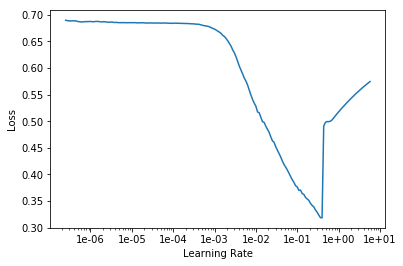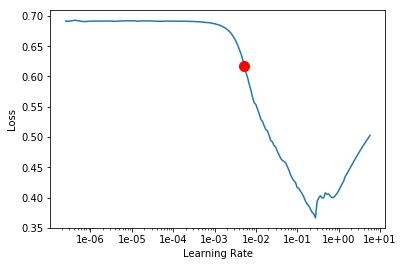Implementation of the LR Range test from Leslie Smith

## Learning Rate Finder¶

Learning rate finder plots lr vs loss relationship for a `Learner`. The idea is to reduce the amount of guesswork on picking a good starting learning rate.

Overview:

1. First run lr_find `learn.lr_find()`
2. Plot the learning rate vs loss `learn.recorder.plot()`
3. Pick a learning rate before it diverges then start training

Technical Details: (first described by Leslie Smith)

Train `Learner` over a few iterations. Start with a very low `start_lr` and change it at each mini-batch until it reaches a very high `end_lr`. `Recorder` will record the loss at each iteration. Plot those losses against the learning rate to find the optimal value before it diverges.

## Choosing a good learning rate¶

For a more intuitive explanation, please check out Sylvain Gugger's post

```path = untar_data(URLs.MNIST_SAMPLE)
data = ImageDataBunch.from_folder(path)
def simple_learner(): return Learner(data, simple_cnn((3,16,16,2)), metrics=[accuracy])
learn = simple_learner()
```

First we run this command to launch the search:

#### `lr_find`[source][test]

`lr_find`(`learn`:`Learner`, `start_lr`:`Floats`=`1e-07`, `end_lr`:`Floats`=`10`, `num_it`:`int`=`100`, `stop_div`:`bool`=`True`, `wd`:`float`=`None`)

Tests found for `lr_find`:

• `pytest -sv tests/test_train.py::test_lr_find` [source]
• `pytest -sv tests/test_vision_train.py::test_lrfind` [source]

To run tests please refer to this guide.

Explore lr from `start_lr` to `end_lr` over `num_it` iterations in `learn`. If `stop_div`, stops when loss diverges.

```learn.lr_find(stop_div=False, num_it=200)
```
```LR Finder is complete, type {learner_name}.recorder.plot() to see the graph.
```

Then we plot the loss versus the learning rates. We're interested in finding a good order of magnitude of learning rate, so we plot with a log scale.

```learn.recorder.plot()
```Then, we choose a value that is approximately in the middle of the sharpest downward slope. This is given as an indication by the LR Finder tool, so let's try 1e-2.

```simple_learner().fit(2, 1e-2)
```
epoch train_loss valid_loss accuracy time
1 0.127434 0.070243 0.973013 00:02
2 0.050703 0.039493 0.984789 00:02

Don't just pick the minimum value from the plot!

```learn = simple_learner()
simple_learner().fit(2, 1e-0)
```
epoch train_loss valid_loss accuracy time
1 0.727221 0.693147 0.495584 00:02
2 0.693826 0.693147 0.495584 00:02

Picking a value before the downward slope results in slow training:

```learn = simple_learner()
simple_learner().fit(2, 1e-3)
```
epoch train_loss valid_loss accuracy time
1 0.152897 0.134366 0.950932 00:02
2 0.120961 0.117550 0.960746 00:02

#### Suggested LR¶

If you pass `suggestion=True` in `learn.recorder.plot`, you will see the point where the gardient is the steepest with a
red dot on the graph. We can use that point as a first guess for an LR.

```learn.lr_find(stop_div=False, num_it=200)
```
```LR Finder is complete, type {learner_name}.recorder.plot() to see the graph.
```
```learn.recorder.plot(suggestion=True)
```
```Min numerical gradient: 5.25E-03
```You can access the corresponding learning rate like this:

```min_grad_lr = learn.recorder.min_grad_lr
```
`0.005248074602497722`
```learn = simple_learner()
```
epoch train_loss valid_loss accuracy time
1 0.109475 0.081607 0.970559 00:02
2 0.070303 0.050977 0.982826 00:02

## `class``LRFinder`[source][test]

`LRFinder`(`learn`:`Learner`, `start_lr`:`float`=`1e-07`, `end_lr`:`float`=`10`, `num_it`:`int`=`100`, `stop_div`:`bool`=`True`) :: `LearnerCallback`

No tests found for `LRFinder`. To contribute a test please refer to this guide and this discussion.

Causes `learn` to go on a mock training from `start_lr` to `end_lr` for `num_it` iterations.

### Callback methods¶

You don't call these yourself - they're called by fastai's `Callback` system automatically to enable the class's functionality.

#### `on_train_begin`[source][test]

`on_train_begin`(`pbar`, **`kwargs`:`Any`)

No tests found for `on_train_begin`. To contribute a test please refer to this guide and this discussion.

Initialize optimizer and learner hyperparameters.

#### `on_batch_end`[source][test]

`on_batch_end`(`iteration`:`int`, `smooth_loss`:`TensorOrNumber`, **`kwargs`:`Any`)

No tests found for `on_batch_end`. To contribute a test please refer to this guide and this discussion.

Determine if loss has runaway and we should stop.

#### `on_epoch_end`[source][test]

`on_epoch_end`(**`kwargs`:`Any`)

No tests found for `on_epoch_end`. To contribute a test please refer to this guide and this discussion.

Called at the end of an epoch.

#### `on_train_end`[source][test]

`on_train_end`(`epoch`:`int`, `num_batch`:`int`, **`kwargs`:`Any`)

No tests found for `on_train_end`. To contribute a test please refer to this guide and this discussion.

Cleanup learn model weights disturbed during LRFinder exploration.